### Nyu?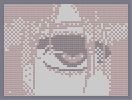Hover over the thumbnail for a full-size version.

Author darestium author:darestium eflen lied n-art nart nyu unrated 2012-08-16 2012-08-16 2 more votes required for a rating. \$Nyu?#darestium#nart#00000000000000000000000000000000000000000000000000000000000000000000000000000000000000000000000000000000000000000000000000000000000000000000000000000000000000000000000000000000000000000000000000000000000000000000000000000000000000000000000000000000000000000000000000000000000000000000000000000000000000000000000000000000000000000000000000000000000000000000000000000000000000000000000000000000000000000000000000000000000000000000000000000000000000000000000000000000000000000000000000000000000000000000000000000000000000000000000000000000000000000000000000000000000000000000000000000000000000000000000000000000000000000000000000000000000000000000000000000000000000000000000000000000000000000000000000000000000000000|12^576,48!12^576,60!12^588,72!12^588,84!12^588,96!12^600,108!12^600,120!12^600,132!12^600,144!12^564,72!12^564,84!12^564,96!12^564,108!12^600,156!12^468,48!12^468,60!12^480,72!12^480,84!12^480,96!12^492,108!12^492,120!12^492,132!12^492,144!12^492,156!12^504,168!12^504,180!12^504,204!12^504,192!12^516,216!12^516,228!12^516,240!12^516,252!12^516,264!12^516,276!12^504,552!12^516,288!12^528,300!12^528,312!12^528,324!12^528,348!12^528,336!12^528,360!12^528,372!12^528,384!12^528,396!12^528,420!12^528,408!12^516,492!12^504,528!12^504,540!12^528,432!12^528,444!12^516,456!12^516,468!12^516,468!12^516,480!12^516,504!12^516,516!12^564,120!12^564,144!12^564,132!12^564,156!12^564,168!12^564,192!12^564,180!12^564,204!12^564,216!12^564,444!12^564,228!12^564,252!12^564,240!12^564,264!12^564,276!12^564,288!12^564,300!12^564,324!12^564,312!12^564,336!12^564,360!12^564,348!12^564,372!12^564,384!12^564,396!12^564,420!12^564,408!12^564,432!12^600,168!12^612,180!12^612,192!12^612,204!12^612,228!12^612,216!12^624,240!12^624,264!12^624,276!12^624,252!12^624,288!12^624,312!12^624,300!12^624,324!12^624,336!12^624,348!12^624,360!12^624,372!12^624,372!12^624,396!12^624,384!12^624,408!12^624,432!12^624,420!12^624,444!12^660,564!12^660,552!12^672,540!12^672,516!12^612,108!12^612,120!12^672,300!12^672,312!12^672,336!12^672,348!12^672,360!12^672,384!12^672,372!12^672,396!12^672,396!12^672,420!12^672,432!12^672,444!12^672,456!12^672,456!12^672,468!12^672,468!12^672,492!12^672,504!12^672,408!12^672,480!12^672,528!12^624,180!12^624,168!12^624,144!12^612,132!12^624,132!12^624,156!12^636,180!12^636,192!12^636,204!12^636,216!12^636,228!12^648,240!12^648,252!12^648,264!12^648,276!12^660,300!12^660,288!12^672,324!12^336,96!12^336,108!12^324,120!12^324,132!12^324,144!12^312,156!12^312,168!12^312,180!12^312,192!12^300,204!12^300,216!12^300,228!12^300,240!12^300,252!12^300,264!12^300,276!12^300,300!12^300,288!12^300,48!12^300,60!12^300,72!12^300,84!12^300,96!12^300,120!12^312,132!12^312,144!12^312,144!12^300,108!12^300,144!12^300,132!12^300,168!12^288,48!12^288,60!12^288,72!12^276,84!12^276,96!12^276,108!12^264,120!12^264,132!12^264,144!12^252,156!12^252,168!12^252,180!12^240,180!12^240,192!12^240,204!12^240,216!12^228,228!12^228,240!12^228,252!12^228,264!12^216,276!12^216,288!12^216,300!12^216,324!12^216,324!12^216,312!12^216,336!12^204,348!12^204,360!12^204,372!12^204,396!12^204,384!12^204,408!12^204,420!12^204,432!12^204,444!12^204,456!12^204,468!12^204,480!12^204,504!12^204,492!12^204,516!12^204,528!12^180,144!12^180,156!12^180,168!12^180,180!12^180,204!12^180,192!12^180,216!12^180,228!12^192,240!12^192,252!12^192,264!12^192,264!12^192,288!12^192,300!12^192,312!12^192,276!12^192,324!12^192,348!12^180,132!12^180,120!12^168,120!12^156,132!12^156,144!12^156,156!12^144,168!12^144,180!12^144,192!12^144,204!12^132,216!12^132,240!12^132,228!12^132,264!12^132,276!12^144,216!12^144,228!12^132,252!12^132,288!12^192,360!12^192,384!12^192,372!12^180,240!12^180,264!12^180,252!12^132,408!12^132,420!10^312,228!10^240,240!10^252,240!10^264,240!10^276,228!10^288,228!10^228,276!10^240,276!10^264,264!10^252,264!10^276,264!10^288,264!10^324,228!10^336,228!10^348,228!10^360,216!10^372,216!10^384,216!10^408,216!10^396,216!10^480,216!10^480,216!10^468,216!10^456,216!10^444,216!10^432,216!10^420,216!10^504,228!10^492,228!10^576,252!10^588,252!10^600,264!10^612,264!10^636,276!10^312,264!10^324,264!10^336,264!10^348,264!10^360,252!10^360,264!10^372,264!10^276,276!10^276,276!10^264,276!10^240,288!10^228,288!10^252,276!10^384,252!10^408,252!10^396,252!10^576,276!10^588,276!10^600,276!10^600,288!10^612,288!10^420,252!10^432,252!10^444,252!10^456,252!10^468,264!10^480,264!10^492,264!10^504,264!10^288,288!10^264,288!10^252,288!10^336,216!10^348,216!10^612,276!10^588,264!10^576,264!10^420,240!10^444,228!10^456,228!10^468,228!10^480,228!10^480,240!10^468,252!10^456,240!10^468,240!10^492,240!10^504,240!10^504,252!10^492,252!10^480,252!10^432,228!10^444,240!10^432,240!10^420,228!10^408,228!10^396,228!10^384,228!10^384,228!10^372,228!10^360,228!10^408,240!10^384,240!10^396,240!10^372,240!10^360,240!10^372,252!10^348,240!10^336,240!10^324,240!10^312,240!10^312,252!10^324,252!10^336,252!10^348,252!10^276,240!10^288,240!10^288,252!10^276,252!10^264,252!10^252,252!10^240,252!10^240,264!10^324,276!10^324,288!10^336,300!10^336,324!10^348,336!10^348,348!10^360,360!10^372,372!10^384,384!10^336,336!10^480,384!10^468,384!10^396,384!10^420,396!10^432,396!10^444,396!10^444,396!10^456,396!10^456,396!10^408,396!10^492,372!10^504,360!10^516,348!10^312,288!10^384,420!10^372,420!10^336,408!10^360,420!10^348,408!10^396,420!10^408,420!10^492,420!10^480,420!10^480,420!10^432,420!10^432,420!10^432,420!10^444,420!10^468,420!10^456,420!10^420,420!10^504,408!10^516,408!10^648,300!10^636,300!10^636,288!10^648,288!10^612,372!10^648,312!10^636,312!10^636,324!10^636,336!10^636,348!10^648,324!10^648,336!10^660,312!10^660,324!10^264,324!10^276,312!10^288,300!10^276,300!10^324,408!10^312,408!10^300,396!10^288,396!10^276,384!12^264,180!12^288,168!12^276,168!10^432,324!10^432,384!10^408,384!10^420,384!10^444,384!10^456,384!10^468,372!10^480,372!10^396,372!10^384,372!10^372,360!10^408,372!10^372,348!10^360,348!10^504,348!10^384,312!10^372,324!10^360,336!10^408,324!10^420,324!10^396,312!10^396,300!10^432,336!10^444,336!10^456,324!10^468,324!10^480,312!10^492,300!10^492,312!10^504,324!10^516,336!10^444,276!10^444,276!10^456,264!10^420,264!10^432,264!10^432,264!10^444,264!10^480,276!10^456,276!10^480,288!10^468,276!10^492,288!10^492,276!10^504,276!10^504,288!10^504,300!10^504,300!10^516,300!10^516,312!10^504,312!10^444,288!10^468,300!10^456,288!10^468,288!10^480,300!10^468,312!10^456,300!10^456,312!10^444,324!10^444,312!10^444,300!10^432,276!10^432,300!10^432,312!10^432,288!10^420,288!10^420,300!10^420,312!10^408,312!10^408,300!10^408,276!10^408,264!10^408,288!10^420,276!10^396,288!10^396,264!10^396,276!10^384,264!10^336,276!10^336,288!10^348,324!10^360,324!10^348,300!10^360,300!10^384,300!10^384,276!10^384,288!10^372,276!10^372,288!10^360,288!10^360,276!10^348,276!10^348,288!10^372,300!10^264,372!10^516,324!12^480,144!12^324,168!12^432,156!12^444,156!12^420,156!12^408,156!12^396,156!12^336,168!12^384,156!12^372,156!12^468,156!12^456,156!12^480,156!12^456,144!12^432,144!12^408,144!12^360,156!12^312,156!12^336,156!12^324,156!12^300,156!12^264,156!12^276,156!12^264,168!12^576,144!12^588,144!12^576,156!12^396,144!12^372,144!10^492,360!10^384,360!12^768,240!12^768,252!12^768,264!12^768,276!12^768,288!12^756,252!12^696,312!12^684,312!12^768,228!12^756,264!12^708,312!12^720,300!12^744,276!12^744,288!12^732,288!12^756,276!12^756,300!12^756,288!12^768,300!12^756,336!12^744,300!12^732,300!12^744,312!12^756,312!12^756,324!12^768,312!12^732,312!12^732,336!12^744,324!12^732,324!12^708,324!12^720,324!12^720,312!12^696,336!12^696,324!12^684,324!12^708,336!12^732,336!12^684,336!12^684,348!12^684,360!12^708,372!12^720,360!12^708,348!12^696,348!12^696,360!12^708,372!12^708,360!12^708,360!12^732,336!12^720,336!12^720,336!12^732,348!12^732,348!12^720,348!12^720,348!12^744,348!12^756,336!12^756,336!12^768,324!12^768,324!12^744,336!12^744,336!12^756,336!12^756,336!12^768,336!12^768,348!12^768,360!12^756,360!12^756,372!12^768,372!12^768,384!12^756,348!12^756,372!12^756,384!12^756,396!12^756,408!12^768,420!12^756,396!12^756,396!12^768,396!12^768,396!12^768,420!12^768,432!12^756,420!12^768,396!12^744,360!12^744,372!12^732,396!12^744,420!12^744,384!12^744,384!12^744,420!12^744,444!12^744,456!12^768,444!12^768,444!12^756,432!12^756,444!12^756,456!12^756,468!12^768,456!12^756,468!12^756,480!12^756,516!12^732,360!12^732,372!12^732,384!12^744,384!12^744,408!12^744,384!12^744,396!12^720,372!12^720,384!12^720,384!12^720,396!12^720,408!12^720,420!12^720,420!12^720,432!12^720,432!12^720,444!12^720,456!12^720,456!12^720,468!12^720,480!12^720,492!12^708,504!12^720,504!12^732,408!12^732,420!12^732,432!12^732,456!12^732,468!12^732,480!12^744,492!12^744,444!12^744,444!12^732,444!12^744,444!12^744,432!12^756,408!12^768,408!12^744,468!12^744,468!12^744,480!12^768,480!12^768,468!12^768,480!12^768,492!12^756,504!12^756,516!12^768,504!12^768,492!12^768,516!12^768,516!12^768,528!12^768,528!12^768,540!12^768,552!12^756,564!12^756,564!12^732,564!12^720,564!12^708,564!12^696,564!12^684,564!12^672,564!12^672,552!12^696,552!12^708,552!12^708,552!12^720,552!12^732,552!12^744,552!12^744,540!12^744,504!12^744,492!12^744,540!12^744,516!12^756,516!12^720,480!12^732,504!12^732,516!12^720,540!12^720,480!12^720,420!12^720,384!12^708,384!12^708,408!12^708,444!12^696,432!12^708,384!12^696,360!12^684,396!12^696,420!12^708,468!12^708,492!12^720,432!12^708,420!12^708,444!12^708,420!12^708,396!12^708,360!12^696,396!12^696,372!12^684,360!12^684,384!12^696,420!12^684,444!12^684,480!12^696,516!12^696,492!12^696,444!12^708,408!12^708,384!12^708,408!12^684,444!12^684,480!12^708,468!12^708,468!12^696,444!12^696,444!12^696,456!12^696,456!12^684,456!12^696,456!12^708,456!12^708,456!12^696,468!12^696,468!12^684,468!12^696,480!12^708,480!12^732,492!12^756,492!12^744,540!12^756,528!12^756,540!12^756,552!12^756,564!12^744,564!12^744,564!12^768,564!12^768,564!12^732,540!12^732,528!12^744,540!12^744,528!12^732,528!12^720,528!12^720,516!12^720,516!12^708,516!12^720,528!12^720,528!12^708,528!12^708,552!12^708,552!12^708,540!12^708,528!12^696,528!12^696,540!12^696,540!12^684,552!12^684,552!12^684,540!12^684,540!12^684,540!12^684,528!12^684,528!12^684,516!12^696,504!12^696,492!12^696,492!12^696,492!12^696,492!12^684,492!12^684,504!12^684,504!12^696,396!12^684,408!12^684,408!12^696,408!12^696,408!12^708,420!12^708,420!12^696,432!12^684,432!12^684,408!12^708,432!12^696,432!12^684,420!12^696,384!12^684,372!12^768,216!12^756,240!12^756,240!12^744,252!12^732,252!12^720,252!12^708,264!12^708,264!12^684,264!12^684,276!12^672,276!12^708,276!12^696,264!12^720,264!12^732,264!12^744,264!12^756,264!12^720,288!12^720,276!12^732,276!12^708,288!12^708,288!12^708,300!12^696,300!12^696,276!12^696,276!12^672,288!12^696,288!12^696,276!12^696,276!12^696,288!12^684,300!12^684,300!12^684,288!12^684,288!12^696,288!12^660,288!12^660,276!12^684,252!12^684,240!12^696,252!12^732,228!12^732,240!12^744,240!12^672,252!12^660,240!12^660,264!12^744,216!12^756,228!12^756,204!12^732,204!12^720,216!12^720,240!12^708,228!12^696,240!12^708,252!12^768,192!12^744,192!12^756,180!12^768,168!12^696,216!12^708,204!12^720,192!12^696,192!12^684,204!12^684,228!12^660,228!12^672,216!12^648,216!12^660,204!12^672,192!12^648,180!12^660,180!12^684,180!12^708,180!12^732,180!12^696,168!12^696,144!12^708,156!12^756,132!12^744,144!12^756,156!12^708,120!12^720,144!12^732,156!12^732,144!12^720,168!12^684,156!12^672,168!12^648,156!12^660,156!12^672,144!12^672,120!12^504,144!12^516,144!12^516,156!12^528,168!12^540,156!12^552,168!12^540,180!12^528,192!12^516,180!12^552,192!12^756,36!12^744,36!12^732,36!12^708,36!12^696,36!12^684,24!12^684,24!12^648,24!12^648,24!12^684,24!12^708,36!12^732,36!12^732,36!12^768,24!12^768,24!12^744,36!12^732,24!12^744,24!12^744,24!12^744,24!12^732,24!12^696,24!12^696,24!12^708,24!12^720,24!12^720,24!12^720,24!12^720,36!12^732,36!12^744,48!12^756,48!12^756,48!12^768,24!12^756,24!12^756,36!12^768,36!12^768,48!12^756,48!12^732,60!12^732,60!12^744,48!12^720,48!12^708,48!12^684,48!12^672,48!12^672,24!12^732,48!12^756,60!12^744,60!12^768,60!12^756,84!12^756,72!12^756,72!12^732,72!12^732,72!12^720,60!12^696,60!12^696,60!12^684,60!12^684,36!12^672,36!12^660,36!12^660,24!12^696,48!12^720,60!12^708,60!12^744,72!12^744,84!12^744,96!12^768,72!12^756,84!12^768,96!12^768,84!12^756,96!12^768,108!12^756,108!12^744,108!12^744,120!12^744,132!12^756,120!12^768,120!12^732,132!12^720,132!12^732,120!12^732,120!12^732,108!12^720,108!12^732,84!12^708,84!12^720,84!12^732,84!12^696,72!12^696,72!12^636,36!12^612,36!12^612,36!12^612,24!12^624,24!12^636,24!12^636,24!12^624,48!12^636,48!12^660,36!12^660,48!12^660,60!12^624,60!12^624,24!12^648,60!12^672,72!12^684,96!12^672,108!12^684,108!12^696,96!12^708,96!12^732,108!12^720,96!12^684,72!12^684,72!12^636,84!12^672,84!12^672,96!12^672,108!12^672,108!12^648,96!12^648,72!12^684,108!12^696,108!12^720,108!12^684,108!12^696,120!12^696,72!12^696,60!12^696,72!12^684,84!12^672,72!12^708,72!12^732,72!12^672,72!12^684,72!12^660,72!12^684,72!12^672,60!12^636,36!12^660,36!12^624,36!12^648,96!12^660,96!12^660,84!12^648,84!12^636,72!12^636,60!12^648,48!12^648,48!12^696,84!12^720,72!12^732,96!12^708,108!12^672,120!12^720,120!12^708,132!12^684,108!12^684,120!12^696,132!12^600,36!12^600,24!12^588,24!12^588,36!12^588,48!12^588,60!12^600,48!12^600,72!12^600,60!12^600,72!12^600,84!12^612,60!12^612,48!12^648,36!12^624,96!12^624,84!12^612,72!12^624,72!12^612,84!12^612,96!12^600,96!12^600,96!12^648,120!12^660,108!12^636,108!12^636,108!12^660,120!12^660,120!12^636,108!12^636,96!12^636,84!12^660,108!12^648,132!12^660,156!12^684,144!12^684,132!12^672,132!12^660,132!12^660,156!12^660,156!12^648,156!12^648,144!12^624,108!12^648,108!12^648,108!12^636,120!12^636,132!12^636,156!12^636,156!12^648,156!12^636,144!12^624,120!12^540,204!12^552,228!12^540,240!12^540,252!12^552,276!12^552,264!12^552,264!12^552,264!12^540,276!12^540,276!12^528,276!12^528,264!12^540,264!12^540,264!12^552,252!12^540,300!12^552,288!12^540,276!12^528,288!12^528,288!12^552,300!12^552,324!12^552,324!12^540,300!12^552,300!12^540,324!12^540,324!12^540,336!12^540,360!12^540,336!12^564,384!12^540,408!12^540,420!12^540,444!12^540,456!12^528,468!12^540,384!12^540,372!12^540,372!12^540,396!12^528,444!12^540,348!12^528,60!12^516,60!12^504,48!12^540,36!12^564,24!12^576,24!12^576,24!12^564,36!12^528,36!12^504,36!12^492,24!12^480,36!12^492,48!12^492,60!12^516,48!12^516,36!12^528,36!12^540,48!12^552,48!12^576,36!12^576,36!12^552,48!12^552,36!12^540,36!12^516,36!12^552,24!12^552,24!12^516,24!12^576,48!12^564,60!12^564,48!12^552,24!12^540,24!12^528,24!12^504,24!12^528,48!12^552,60!12^540,60!12^552,72!12^540,84!12^540,72!12^528,72!12^504,72!12^504,60!12^492,48!12^480,60!12^480,36!12^492,36!12^480,48!12^516,72!12^540,72!12^528,72!12^516,72!12^516,84!12^528,84!12^540,72!12^552,72!12^564,84!12^552,96!12^552,96!12^552,84!12^552,84!12^564,108!12^552,120!12^552,120!12^540,108!12^552,108!12^552,120!12^528,132!12^516,120!12^516,96!12^528,96!12^540,108!12^540,108!12^540,96!12^540,108!12^540,120!12^552,132!12^540,132!12^516,120!12^516,96!12^516,96!12^504,96!12^504,84!12^492,84!12^492,84!12^492,72!12^492,96!12^516,96!12^516,108!12^540,108!12^540,120!12^504,120!12^528,108!12^516,120!12^504,132!12^552,144!12^528,144!12^528,456!12^360,24!12^360,36!12^348,84!12^480,24!12^468,24!12^456,36!12^456,24!12^468,36!12^300,180!12^300,192!12^348,48!12^348,60!12^336,72!12^336,84!12^348,72!12^348,24!12^336,48!12^336,60!12^348,36!12^324,36!12^312,36!12^312,36!12^312,48!12^312,60!12^312,60!12^312,72!12^312,84!12^312,108!12^324,96!12^324,72!12^132,432!12^120,444!12^132,444!12^132,504!12^120,456!12^120,480!12^120,480!12^120,492!12^108,468!12^120,468!12^120,504!12^132,516!12^120,516!12^132,528!12^132,540!12^132,552!12^108,372!12^108,408!12^120,372!12^120,372!12^120,408!12^108,432!12^108,396!12^132,420!12^108,420!12^108,384!12^108,444!12^96,420!12^36,372!12^60,444!12^60,444!12^72,432!12^60,360!12^108,444!12^108,396!12^96,372!12^84,360!12^60,408!12^60,408!12^48,360!12^60,360!12^60,372!12^60,384!12^72,396!12^84,396!12^84,384!12^60,360!12^72,360!12^84,372!12^84,408!12^84,408!12^84,432!12^72,444!12^72,384!12^96,408!12^96,396!12^96,396!12^96,384!12^132,408!12^108,408!12^108,408!12^108,396!12^120,396!12^132,432!12^120,420!12^84,480!12^72,504!12^48,492!12^108,456!12^108,456!12^96,432!12^96,456!12^96,444!12^96,444!12^96,480!12^108,480!12^108,444!12^120,420!12^108,444!12^108,432!12^120,432!12^120,504!12^108,492!12^108,456!12^120,504!12^120,516!12^120,540!12^108,552!12^96,564!12^72,540!12^48,468!12^48,432!12^84,480!12^84,480!12^84,456!12^72,420!12^60,372!12^84,456!12^84,480!12^84,444!12^84,408!12^96,516!12^84,492!12^72,456!12^96,468!12^96,456!12^72,468!12^60,432!12^72,384!12^72,408!12^60,468!12^60,408!12^60,396!12^36,384!12^36,384!12^60,384!12^60,384!12^72,372!12^72,372!12^36,372!12^48,384!12^48,372!12^48,372!12^48,420!12^48,420!12^36,420!12^36,432!12^60,420!12^60,420!12^96,420!12^84,420!12^48,396!12^48,396!12^24,396!12^36,396!12^36,396!12^36,420!12^48,408!12^60,408!12^24,408!12^36,408!12^36,408!12^36,432!12^24,420!12^24,420!12^24,480!12^36,456!12^60,492!12^60,468!12^48,432!12^84,468!12^84,516!12^84,492!12^108,492!12^108,516!12^108,516!12^108,516!12^96,504!12^96,516!12^96,492!12^96,492!12^96,516!12^96,504!12^96,504!12^108,504!12^108,528!12^120,564!12^120,576!12^120,576!12^132,564!12^132,576!12^36,552!12^36,516!12^60,468!12^84,492!12^72,492!12^60,480!12^36,408!12^36,408!12^12,504!12^24,528!12^48,552!12^36,528!12^48,468!12^60,456!12^72,492!12^84,492!12^72,468!12^72,480!12^84,516!12^72,528!12^60,504!12^36,456!12^36,432!12^60,456!12^48,444!12^48,444!12^36,432!12^36,432!12^36,444!12^36,444!12^36,456!12^36,456!12^36,480!12^48,492!12^48,468!12^48,456!12^48,456!12^36,480!12^36,480!12^36,456!12^24,432!12^36,444!12^24,468!12^36,444!12^84,504!12^72,516!12^60,528!12^60,504!12^60,492!12^36,468!12^24,456!12^24,432!12^24,444!12^48,492!12^48,480!12^48,492!12^48,504!12^48,516!12^60,516!12^60,516!12^60,528!12^60,528!12^60,528!12^72,540!12^84,540!12^96,528!12^84,528!12^120,528!12^132,528!12^120,528!12^108,552!12^108,552!12^108,576!12^120,552!12^120,552!12^108,564!12^108,564!12^120,540!12^84,540!12^96,552!12^96,552!12^96,540!12^84,552!12^96,576!12^96,576!12^84,576!12^84,576!12^60,564!12^48,576!12^48,576!12^48,576!12^36,576!12^24,564!12^36,504!12^36,492!12^24,492!12^24,504!12^24,516!12^48,540!12^48,528!12^60,540!12^72,552!12^84,552!12^72,564!12^84,564!12^72,576!12^72,576!12^60,576!12^36,564!12^48,564!12^36,576!12^24,576!12^36,540!12^24,540!12^24,552!12^108,540!12^60,300!12^60,288!12^72,288!12^72,288!12^60,276!12^60,276!12^72,276!12^72,276!12^84,276!12^96,264!12^96,264!12^96,264!12^84,276!12^96,276!12^108,276!12^108,300!12^96,312!12^96,324!12^96,312!12^96,300!12^120,300!12^120,336!12^120,312!12^132,300!12^96,360!12^84,336!12^84,324!12^36,372!12^60,372!12^84,348!12^96,348!12^108,360!12^108,360!12^120,348!12^108,348!12^96,336!12^108,312!12^108,312!12^108,336!12^108,336!12^96,312!12^108,324!12^384,324!12^384,348!12^372,336!12^396,336!12^420,336!12^408,348!12^420,360!12^432,372!12^432,348!12^444,360!12^456,348!12^456,372!12^468,336!12^480,348!12^468,360!12^492,324!12^504,336!12^84,300!12^108,288!12^108,288!12^96,288!12^96,288!12^72,312!12^60,324!12^60,336!12^84,336!12^72,348!12^72,336!12^84,324!12^84,300!12^84,300!12^72,288!12^72,300!12^72,312!12^72,324!12^96,324!12^84,312!12^84,312!12^84,288!12^72,312!12^36,336!12^24,300!12^36,324!12^48,312!12^48,348!12^36,360!12^36,348!12^72,324!12^72,324!12^60,300!12^36,348!12^36,312!12^36,312!12^60,312!12^60,300!12^36,348!12^36,348!12^48,336!12^48,324!12^48,336!12^60,348!12^36,312!12^24,324!12^24,312!12^36,324!12^24,336!12^24,348!12^24,372!12^24,372!12^48,288!12^24,288!12^36,276!12^24,264!12^48,264!12^60,252!12^72,264!12^84,252!12^120,264!12^120,288!12^108,252!12^36,252!12^24,240!12^24,216!12^24,192!12^24,168!12^24,144!12^36,156!12^36,180!12^36,204!12^36,228!12^48,240!12^48,216!12^48,192!12^60,180!12^60,204!12^60,228!12^72,240!12^72,216!12^84,228!12^84,204!12^72,192!12^84,180!12^96,240!12^96,216!12^108,228!12^120,240!12^96,192!12^108,204!12^48,168!12^120,216!12^120,192!12^108,180!12^96,168!12^108,156!12^120,168!12^132,156!12^84,156!12^96,144!12^120,144!12^108,132!12^132,132!12^120,120!12^96,120!12^72,168!12^108,108!12^132,108!12^336,24!12^312,24!12^276,24!12^264,24!12^240,36!12^228,36!12^204,36!12^180,36!12^156,36!12^132,24!12^120,36!12^132,48!12^240,48!12^264,48!12^288,36!12^288,36!12^264,24!12^264,24!12^300,36!12^264,24!12^288,36!12^300,24!12^300,24!12^288,24!12^276,36!12^264,48!12^252,48!12^264,36!12^264,36!12^228,24!12^228,24!12^204,36!12^168,36!12^144,36!12^108,24!12^108,24!12^72,48!12^228,36!12^192,36!12^192,36!12^216,36!12^204,24!12^204,24!12^180,24!12^168,24!12^168,24!12^144,24!12^144,24!12^204,24!12^204,24!12^204,24!12^192,24!12^252,24!12^252,36!12^228,24!12^240,24!12^216,48!12^204,48!12^192,48!12^192,48!12^180,48!12^180,48!12^168,48!12^156,48!12^192,36!12^228,48!12^216,36!12^216,24!12^120,24!12^156,24!12^132,36!12^144,48!12^216,48!12^192,60!12^168,72!12^144,60!12^192,48!12^180,60!12^168,72!12^168,72!12^168,60!12^156,60!12^156,72!12^168,84!12^228,60!12^240,60!12^276,48!12^276,48!12^276,48!12^276,60!12^276,60!12^252,60!12^252,60!12^264,72!12^276,72!12^264,72!12^252,72!12^276,48!12^276,48!12^120,48!12^96,48!12^96,36!12^108,36!12^108,36!12^108,48!12^84,24!12^60,36!12^60,36!12^24,48!12^60,72!12^60,36!12^72,36!12^84,48!12^84,36!12^96,36!12^108,24!12^72,36!12^108,24!12^96,24!12^132,60!12^120,48!12^120,60!12^120,60!12^96,60!12^60,72!12^60,72!12^48,60!12^48,48!12^84,60!12^84,60!12^108,60!12^108,60!12^72,48!12^72,60!12^72,60!12^60,48!12^72,36!12^72,24!12^48,24!12^48,60!12^84,48!12^60,24!12^60,48!12^72,48!12^60,36!12^60,36!12^36,36!12^36,36!12^48,36!12^36,24!12^36,36!12^24,24!12^36,36!12^24,36!12^36,48!12^36,60!12^36,60!12^36,72!12^36,84!12^36,84!12^36,96!12^36,120!12^36,108!12^36,60!12^24,60!12^24,84!12^24,84!12^24,108!12^24,108!12^36,120!12^24,72!12^24,96!12^24,120!12^36,72!12^60,60!12^204,60!12^216,60!12^264,60!12^144,72!12^132,72!12^120,72!12^108,72!12^108,72!12^96,72!12^84,72!12^72,72!12^48,72!12^48,84!12^48,96!12^48,108!12^48,108!12^48,120!12^48,132!12^48,132!12^36,132!12^240,72!12^228,72!12^216,72!12^204,72!12^204,72!12^192,72!12^180,72!12^132,84!12^108,84!12^96,84!12^84,84!12^84,84!12^84,96!12^72,96!12^72,84!12^72,84!12^48,96!12^60,96!12^60,120!12^72,120!12^72,108!12^72,96!12^60,96!12^60,84!12^72,84!12^60,144!12^72,156!12^60,156!12^48,156!12^48,144!12^48,132!12^24,132!12^48,132!12^60,120!12^72,108!12^84,108!12^84,108!12^84,132!12^72,132!12^60,132!12^72,108!12^72,108!12^60,108!12^144,84!12^120,96!12^96,108!12^120,84!12^144,72!12^192,72!12^204,144!12^192,156!12^204,168!12^192,180!12^204,192!12^192,204!12^216,156!12^216,180!12^216,204!12^216,216!12^228,216!12^228,216!12^228,204!12^216,216!12^204,228!12^204,216!12^204,216!12^180,228!12^192,216!12^204,228!12^216,228!12^216,228!12^204,264!12^204,264!12^204,252!12^216,240!12^216,252!12^204,264!12^204,264!12^204,276!12^192,300!12^204,300!12^192,324!12^192,336!12^192,324!12^204,300!12^204,312!12^204,324!12^204,324!12^204,276!12^228,84!12^228,84!12^264,84!12^264,84!12^264,84!12^252,84!12^252,84!12^240,84!12^252,108!12^252,108!12^228,108!12^192,108!12^204,84!12^204,84!12^228,84!12^240,108!12^204,108!12^300,108!12^252,96!12^264,120!12^276,120!12^288,120!12^288,96!12^300,84!12^300,84!12^324,96!12^360,144!12^288,84!12^192,84!12^180,84!12^216,84!12^156,84!12^108,96!12^96,96!12^144,96!12^192,96!12^168,96!12^156,96!12^180,108!12^180,108!12^156,108!12^156,120!12^156,120!12^132,96!12^120,96!12^216,96!12^228,96!12^240,96!12^240,96!12^216,96!12^204,96!12^180,96!12^168,108!12^168,108!12^144,108!12^144,120!12^144,120!12^144,144!12^144,144!12^132,180!12^132,192!12^132,192!12^144,552!12^144,564!12^144,576!12^120,324!12^120,360!12^120,384!12^132,468!12^132,456!12^132,468!12^132,480!12^132,492!12^120,276!12^24,384!12^24,360!12^420,372!12^444,372!12^480,360!12^408,360!12^408,360!12^408,360!12^396,360!12^396,324!12^480,324!12^480,324!12^456,336!12^408,336!12^396,348!12^432,360!12^468,348!12^492,348!12^480,336!12^456,360!12^480,132!12^456,132!12^456,132!12^468,132!10^612,300!10^588,300!10^600,312!10^588,324!10^612,324!10^600,336!10^612,348!10^600,360!10^576,312!10^576,288!10^588,348!10^588,372!10^600,372!12^348,168!12^360,156!12^372,156!12^396,168!12^396,168!12^372,168!12^372,168!12^384,168!12^360,168!12^348,144!12^324,144!12^336,144!12^444,132!12^432,132!12^420,132!12^420,132!12^396,132!12^384,132!12^408,132!12^372,132!12^576,132!12^588,144!12^588,132!12^420,204!12^396,204!12^372,204!12^384,192!12^396,192!12^408,192!12^360,192!12^348,204!12^336,192!12^324,204!12^324,216!12^288,204!12^276,216!12^252,204!12^264,204!12^264,216!12^252,228!12^432,192!12^444,204!12^456,192!12^432,204!12^456,192!12^468,204!12^480,204!12^492,204!12^504,216!12^588,168!12^576,180!12^588,192!12^576,204!12^600,204!12^588,216!12^600,228!12^576,228!12^588,240!12^612,252!12^600,240!12^576,84!12^576,108!12^588,120!12^420,432!12^408,444!12^420,456!12^432,444!12^444,432!12^456,444!12^468,432!12^480,444!12^492,432!12^504,420!12^516,432!12^276,396!12^288,408!12^300,420!12^324,420!12^336,432!12^348,420!12^360,432!12^372,444!12^384,432!12^396,444!12^348,432!12^480,432!12^300,408!12^420,444!12^432,36!12^444,48!12^444,24!12^456,72!12^288,132!12^276,144!12^144,492!12^156,504!12^144,516!12^168,516!12^156,528!12^168,540!12^144,540!12^156,552!12^168,552!12^156,564!12^180,564!12^180,528!12^192,540!12^192,552!12^204,564!12^192,576!12^168,576!12^144,396!12^156,384!12^168,384!12^180,372!12^240,348!12^228,348!12^132,384!12^144,372!12^156,360!12^168,372!12^132,360!12^144,348!12^132,336!12^156,336!12^180,336!12^168,348!12^180,360!12^168,144!12^168,168!12^156,180!12^168,192!12^156,204!12^168,216!12^156,228!12^168,240!12^144,240!12^156,252!12^168,264!12^144,264!12^156,276!12^180,276!12^144,288!12^168,288!12^156,300!12^180,300!12^168,312!12^144,312!12^156,324!12^180,324!12^132,324!12^204,120!12^216,108!12^276,96!12^276,96!12^264,96!12^264,108!12^240,120!12^240,120!12^228,120!12^228,120!12^264,132!12^264,132!12^240,120!12^252,108!12^252,132!12^228,144!12^216,144!12^204,120!12^216,120!12^252,144!12^228,120!12^228,120!12^240,132!12^228,132!12^216,132!12^204,120!12^228,156!12^228,156!12^240,168!12^240,168!12^216,132!12^204,120!12^180,120!12^180,408!12^180,444!12^180,480!12^156,420!12^156,456!12^192,132!12^192,120!12^660,348!12^648,360!12^660,372!12^648,384!12^660,396!12^648,408!12^660,420!12^648,432!12^636,372!12^636,396!12^636,420!12^660,444!12^600,384!12^612,396!12^648,456!12^660,480!12^588,396!12^600,408!12^636,444!12^648,468!12^660,504!12^576,408!12^588,420!12^612,420!12^660,528!12^324,324!12^312,312!12^324,300!12^300,324!12^660,576!12^672,576!12^684,576!12^696,576!12^768,132!12^768,576!12^756,576!12^744,576!12^732,576!12^720,576!12^708,576# My first 'N' art. Hope you like it.

## Other maps by this author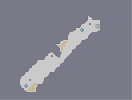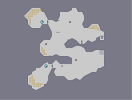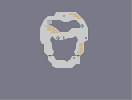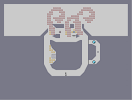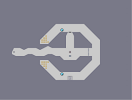Sloped Attenuation Guarded Catacombs Fukkit Bukkit Slurp it up! Octcel Chase Three gram rocks

Pages: (0)

### and

Mrygy05, dragonmoon are amazing artists

### Stuffed up my BBCode, I suck.

Good N-Artists:

~courtesy of Sunset [nmaps.net]

### I like this alot!

Great for you first N-Art! Only took you 2 hours as well. :P
BluePretzel, I told him about z-snapping because he didn't know about it, that will make him a better n-artist.
Good N-Artists:

~courtesy of Sunset~
[nmaps.net]

### make more

i miss good nartists

### It's nice...

But try looking into some good n-artists, like iban, espada, and KNO3 (or whatever, forgot his name). You're good, just not to their amazing standard. I suppose that can be taken as an insult, so I apologize in advance.

### This is probably...

on of the BEST n-arts I've seen in a while!!
The eye part looks pretty neat. The rest feels a bit meh, but that might just be me.

### Ugh

Missed that spot in the left hand corner, damn text :(